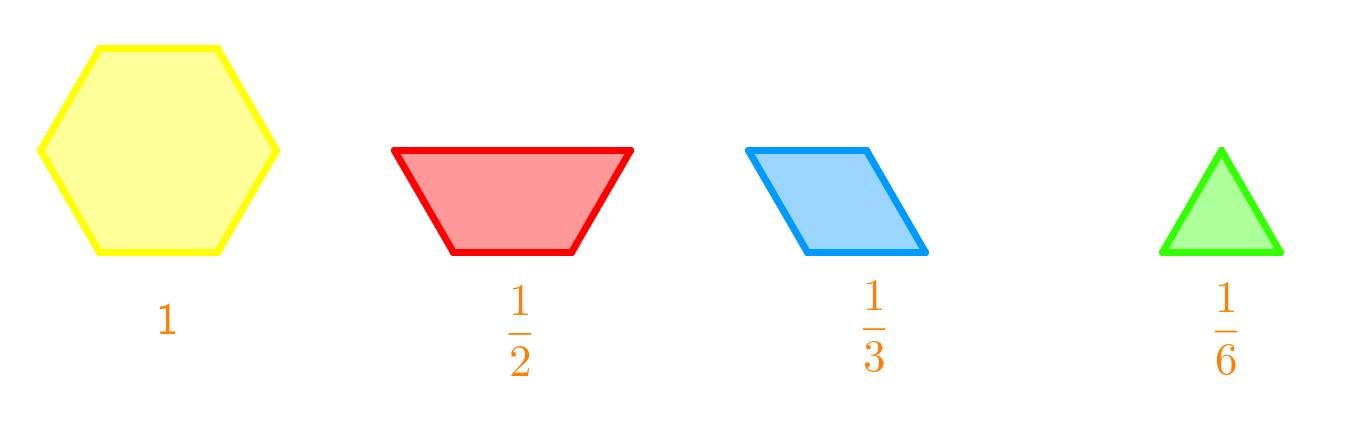# Adding and subtracting mixed numbers#### All You Need in One Place

Everything you need for better marks in primary, GCSE, and A-level classes.#### Learn with Confidence

We’ve mastered the UK’s national curriculum so you can study with confidence.#### Instant and Unlimited Help

0/6
##### Intros
###### Lessons
1. Simplify fractions: Method A - By using greatest common factors
2. Simplify fractions: Method B - By using common factors
3. What are equivalent fractions?
5. How to subtract frations?
• subtracting with improper fractions
• subtracting with mixed numbers
6. Proper fractions VS. Improper fractions VS. Mixed fractions
0/5
##### Examples
###### Lessons
1. Subtract or add using the given diagram.1.2.1. $1\frac{3}{6}+\frac{3}{8}$
3. Maren goes to soccer practice for $2\frac{4}{5}$ hour each day. In the morning, she has $1\frac{3}{4}$ hour of practice. How many hours of practice does she have in the afternoon?
1. Hannah ran for $1\frac{1}{4}$ hour and then biked for $1\frac{2}{3}$ hour. For how long did she exercise?